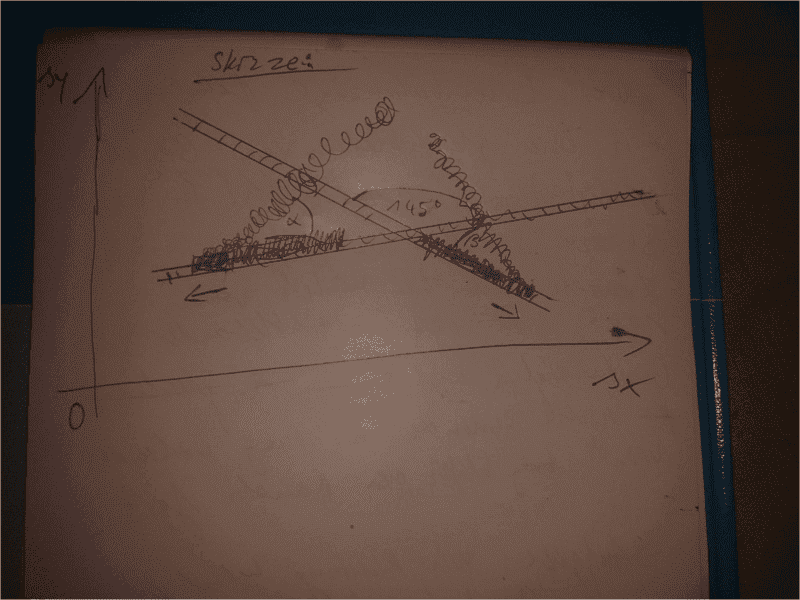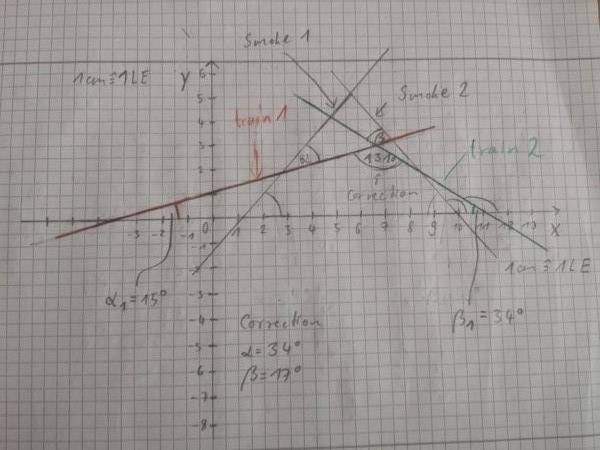# How to figure out the velocity and direction of the wind

• orpheus
This is not complicated. I do not understand why you are having trouble with it. It is a simple trigonometric equation. If you are having trouble with it, I suggest you study trigonometry.f

## Homework Statement

[/B]
So here is my question: I asked you about a solution approach/solution for the following task: Two rail paths cross at an angle of 145 °. With a constant speed of 60 km/h, they just drive along their tracks, and each of them repels steam/smoke flags, which, among other things, are deflected by a wind that does not change its direction and keeps its speed. Be. Order is the determination of the direction or vector coordinates and the velocity of the wind.

See (sketch): https://share-your-photo.com/12ca4a26fe## Homework Equations

depending to the sketch:

α=36°

β=18°

## The Attempt at a Solution

[/B]
My ideas: I know that all vectors are komplanar to each other because they are all on one level (r ^ 2). Accordingly, each vector can also be represented as a linear combination of the other.
So my idea in this direction went to determine the searched vector by means of this method, but the coefficients are unknown. How to solve it by construction is unclear to me (the picture of me is supposed to be accepted as scale). The speed could sometimes be determined by the Fahrrwind = Speed trains. I have no detailed idea of a solution regarding this problem, which is why I ask for help.

#### Attachments

Last edited by a moderator:

First, I ask myself, what do we assume the smoke would do in the absence of wind? I assume it would be growing directly behind the train at a rate of 60 km/h. So that would be a vector pointing in the opposite direction to the train, magnitude 60 km/h. I'll say the vectors ##\vec s_1## and ##\vec s_2## of this undisturbed smoke have components ##s_{1x}## and ##s_{1y}## for train 1, and ##s_{2x}## and ##s_{2y}## for train 2.

The observed direction is a vector sum of that vector and the wind vector ##\vec w##. Let us call its components ##w_x## and ##w_y##.

Finally, we'll use ##\vec v_1## and ##\vec v_2## for the resultant vectors with components ##v_{1x} = s_{1x} + w_x##, ##v_{2x} = s_{2x} + w_x## and similar for ##y##.

I cannot tell from your picture what the exact direction of each train is. I will assume the first train is moving in the -x direction. So the smoke is growing in the +x direction with no wind, i.e., ##s_{1x} = 60## and ##s_{1y} = 0##. With the wind added, we have
$$v_{1x} = 60 + w_x$$
$$v_{1y} = w_y$$
and the direction of this vector is ##\alpha## relative to the +x axis, so
$$\tan(\alpha) = \frac{v_{1y}}{v_{1x}} = \frac{w_y}{60 + w_x}$$

Now for train number 2. ##\vec s_2## is at an angle of ##145^\circ## relative to the +x axis, so ##s_{1x} = 60 \cos(145^\circ)## and ##s_{1y} = 60 \sin(145^\circ)##. Thus you can form the expressions for ##v_{2x}, v_{2y},## and ##\tan(\beta)##.

End result: two equations for ##w_x## and ##w_y## which are easily rearranged into linear equations you can solve.

Thank you so far, I'm not quite sure what it would look like when I print for tan beta. Above that one has two partial speeds, almost one of these simply over the Pythagorean theorem to a set together?

*the different velocities

Excuse me for the picture. Train number 1 isn't moving parallely to x-axis. So is it right that s_1_y should be 0?

The triangles in the sketch by the Rachfahnen are not perpendicular, you can not use angular functions like tan?

Thank you so far, I'm not quite sure what it would look like when I print for tan beta. Above that one has two partial speeds, almost one of these simply over the Pythagorean theorem to a set together?

I'm sorry, I do not understand what you are asking here.

Train number 1 isn't moving parallely to x-axis. So is it right that s_1_y should be 0?
No, if it is moving at an angle to the x axis, then the y component is nonzero. As I said, I'm guessing. I'm making assumptions.

If it is not moving parallel to the x axis, then we are back to the situation where we do not know which direction either train is moving in the (x,y)-plane, therefore we can not define the direction of the wind. Unless you were given some direction information which for some reason you omitted.

The triangles in the sketch by the Rachfahnen are not perpendicular, you can not use angular functions like tan?
For any vector its direction ##\theta##, defined as the angle relative to the +x axis, is related to its components ##v_x## and ##v_y## by ##\tan(\theta) = v_y/v_x## (so long as ##v_x \neq 0##). That holds for every vector in this problem.

What I was attempting to do was work out equations for the x and y components of trains, wind, and direction of smoke. Your diagrams are not showing x and y components. You know how to find the x and y components of a vector given its direction, right?

The second equation I alluded to was to figure out what the angle of the second train's smoke is, relative to the x axis. I was mistaken, that won't be ##\beta##. But it will be some expression in terms of ##\beta##. And as usual, its tangent will be the ratio of y component to x component.

Unfortunately the equations for x and y components were based on knowing the directions of the trains. Now you tell me we don't know that.

Sorry, I have informed myself again about the task and so the angles are corrected. The differences are not very much, the relations are still the same. Also the directions of both trains are given with angles and there is a x and y axis. Excuse me for some cases I just used in some cases google translator. :D#### Attachments

Last edited by a moderator: